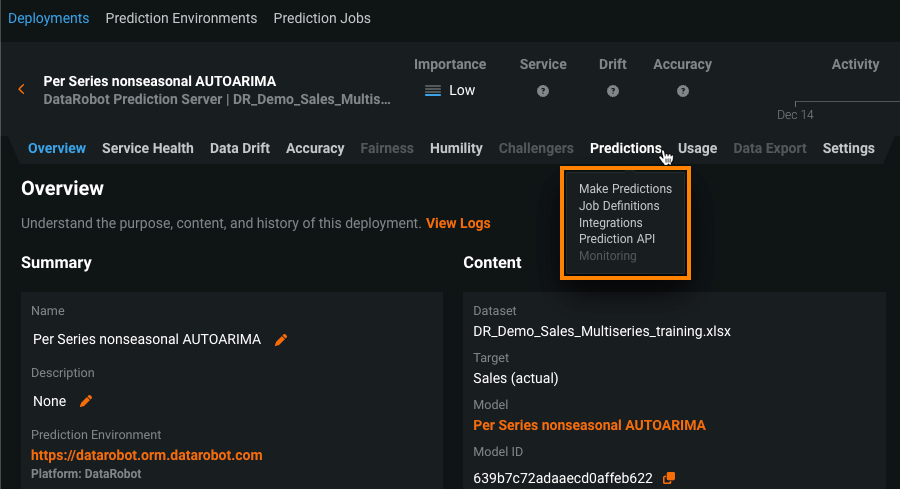Click in-app to access the full platform documentation for your version of DataRobot.

# Batch predictions for TTS and LSTM models¶

Availability information

Batch predictions for TTS and LSTM models, available as a public preview feature, are off by default. Contact your DataRobot representative or administrator for information on enabling the feature.

Feature flag: Enable TTS and LSTM Time Series Model Batch Predictions

Traditional Time Series (TTS) and Long Short-Term Memory (LSTM) models— sequence models that use autoregressive (AR) and moving average (MA) methods—are common in time series forecasting. Both AR and MA models typically require a complete history of past forecasts to make predictions. In contrast, other time series models only require a single row after feature derivation to make predictions. Previously, batch predictions couldn't accept historical data beyond the effective feature derivation window (FDW) if the history exceeded the maximum size of each batch, while sequence models required complete historical data beyond the FDW. These requirements made sequence models incompatible with batch predictions. Enabling this public preview feature removes those limitations to allow batch predictions for TTS and LSTM models.

Note

Time series Autopilot still doesn't include TTS or LSTM model blueprints; however, you can access the model blueprints in the model Repository.

To allow batch predictions with TTS and LSTM models, this feature:

• Updates batch predictions to accept historical data up to the maximum batch size (equal to 50MB or approximately a million rows of historical data).

• Updates TTS models to allow refitting on an incomplete history (if the complete history isn't provided).

Warning

If you don't provide sufficient historical data at prediction time, you could encounter prediction inconsistencies. For more information on maintaining accuracy in TTS and LSTM models, see the prediction accuracy considerations below.

Without this feature enabled, the Predictions > Make Predictions and Predictions > Job Definitions tabs are unavailable for TTS and LSTM models.With this feature enabled, you can access the Predictions > Make Predictions and Predictions > Job Definitions tabs of a deployed TTS or LSTM model.

What models are impacted by this change?

The following model types can now make batch predictions:

• Recurrent Neural Network Regressor using Keras
• Recurrent Neural Network Regressor using Keras for each forecast distance
• Univariate Recurrent Neural Network Regressor using Keras
• Multi-Step Recurrent Neural Network Regressor using Keras
• Sequence to sequence Recurrent Neural Network Regressor using Keras
• DeepAR Recurrent Neural Network Regressor using Keras
• Seasonal AUTOARIMA model based on statsmodels SARIMAX model
• Nonseasonal AUTOARIMA model based on statsmodels SARIMAX model
• Per Series Nonseasonal AUTOARIMA model based on statsmodels SARIMAX model
• AUTOARIMA model based on statsmodels SARIMAX model using baseline offset for each forecast distance
• Nonseasonal AUTOARIMA model based on statsmodels SARIMAX model using Fourier Terms
• Per-series Nonseasonal AUTOARIMA model based on statsmodels SARIMAX model using Fourier Terms
• Error-Trend-Seasonal (ETS) exponential smoothing model
• Per-series AutoETS model based on statsmodels ETS model
• TBATS forecasting regressor
• Prophet
• Per-series Prophet
• Vector Autoregressive Model (VAR)
• VARMAX model based on stats model (Multiseries VARMAX model)
• Multiseries VARMAX model with Fourier features
• Per-series TBATS forecasting regressor

## Prediction accuracy considerations¶

Calculating the percentage difference in RMSE between "full history" and "incomplete history" predictions measures the impact of using an incomplete feature derivation window history when making batch predictions with sequence models. Based on testing, DataRobot recommends applying the following guidelines to maintain prediction accuracy:

• ARIMA and ETS: These models use a smooth method (based on Kalman filtering), which does not change model parameters and uses the original model if refitting fails. To maintain accuracy, provide at least 20 points of historical data. It is particularly important to provide sufficient historical data to effectively smooth the new data with existing parameters when the FDW is small and the forecast isn't seasonal.

• TBATS and PROPHET: These models use a warm-start method, which uses the existing model parameters as an initial "guess" and completes the refit with more data. The model parameters can change, and the accuracy results are less consistent. To maintain accuracy, provide at least 40 points of historical data.

• LSTM: There are two groups of LSTM models.

• The following models have the same requirements at prediction time as most time series models—prediction data must contain an effective feature derivation window history. These models are:

• Recurrent Neural Network Regressor using Keras
• Recurrent Neural Network Regressor using Keras for each forecast distance
• The following models require additional history to ensure accurate and consistent predictions for all prediction methods. While they will return predictions if historical data allows for effective feature derivation window size, the most accurate predictions require additional historical data rows. The amount can be calculated as:

`2 * (feature derivation window start + forecast window end + 1)`.

For example, if features are derived on a [7, 0] days interval and forecast at [2,5] days, the amount of history required is `2 * [7 + 5 + 1]`, or 26 days.

These models are:

• Univariate Recurrent Neural Network Regressor using Keras
• Multi-Step Recurrent Neural Network Regressor using Keras
• Sequence to Sequence Recurrent Neural Network Regressor using Keras (LSTM)
• DeepAR Recurrent Neural Network Regressor using Keras

Updated March 21, 2023